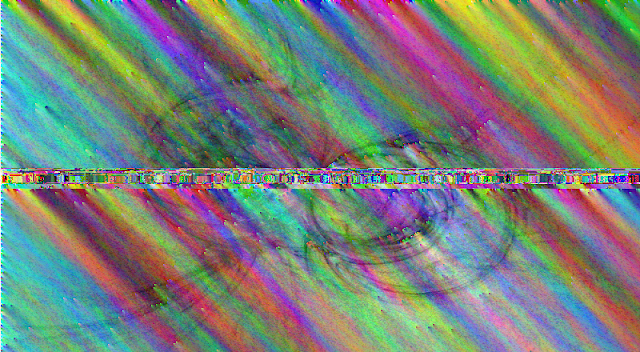# Лаборатория→ TJCTF 2016 - corruption [forensics 130]

, 01 June 2016

Have a free flag! It might have gotten corrupted in transmission, but that shouldn't be a problem for you. Right?

Corrupted PNG file is given.
No width, height, bit depth, color type in IHDR chunk, all IDAT chunks' lengths are currupted too.

I've decided to decode each IDAT chunks and save as BMP :)
My teammate Progressor also was solving this task, but in another way.
He fixed all chunks and got the next image:But it is hard to see the flag here.
Here is my code to decode and save all IDAT chunks to BMP:

```import zlib
import struct

d = zlib.decompressobj()

with open('corrupted_a73df730c202ea0aa636bb607cf52a98d40462f15447927085bb948495e1b187.png', 'rb') as f:

num = 0
all_data = ''

while True:
idat_pos = data.find('IDAT')
if idat_pos < 0:
break
data = data[idat_pos + 4:]
all_data += data[:8200 - 8]
num += 1

image_width = 800 - 1
image_height = 440
bits_per_pixel = 24

bmp_data = 'BM'
bmp_data += struct.pack('i', 26 + bits_per_pixel / 8 * image_width * image_height)
bmp_data += struct.pack('h', 0) # reserved
bmp_data += struct.pack('h', 0) # reserved
bmp_data += struct.pack('i', 26) # offset

bmp_data += struct.pack('i', 12) # size of this header (12 bytes)
bmp_data += struct.pack('h', image_width) # width
bmp_data += struct.pack('h', image_height) # height
bmp_data += struct.pack('h', 1) # number of color planes, must be 1
bmp_data += struct.pack('h', bits_per_pixel) # bits per pixel

try:
dec = d.decompress(all_data[:-10])
bmp_data += ''.join(x if ord(x) < 200 else '\x00' for x in dec[::1])
except Exception as ex:
print ex

with open('corrupt.bmp', 'wb') as f:
f.write(bmp_data)
```

Width and height values were found experimentally.

My file after vertical flip:After some filtering of image I've discerned a flag:
tjctf{c0rruption? wh4t corrupt10n?}